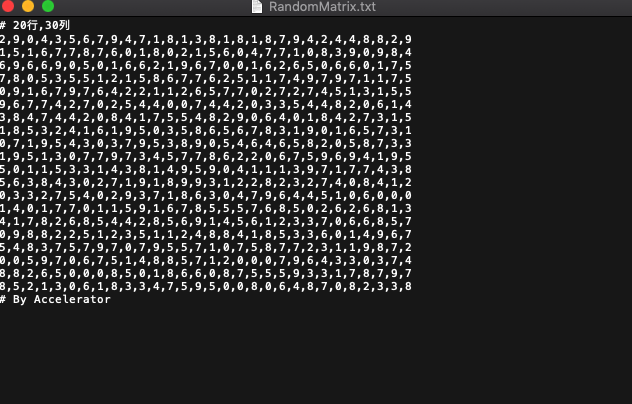• python3 的 random库里面有一个函数，方便的有点吓人。 &gt;&gt;&gt; help(random.choice) Help on method choice in module random: choice(seq) method of random.Random instance Choose a random...
python3 的 random库里面有一个函数，方便的有点吓人。

>>> help(random.choice)
Help on method choice in module random:

choice(seq) method of random.Random instance
Choose a random element from a non-empty sequence.

它的作用是传入一个可迭代的、有index的东西也就是列表和tuple，返回给你其中任意一个的数据。

random.choice(lista)

这样省去了广大懒人编写随机返回库的时间。不过注意，函数不会注意到前面的数据已经被选择输出了。刚刚输出的，也可能立刻再被输出一次。

展开全文random
• python中的numpy.random.randint(a,b)用于生成一个指定范围内的c行d列的整数矩阵。其中参数a是下限，参数b是上限，生成的随机数矩阵n: a <= n <= b。前提是必须import numpy，如果直接import random后调用...
注释

在python中的numpy.random.randint(a,b)用于生成一个指定范围内的c行d列的整数矩阵。其中参数a是下限，参数b是上限，生成的随机数矩阵n: a <= n <= b。前提是必须import numpy，如果直接import random后调用random.randint()并且尝试输入size1参数，会出现以下错误：
Traceback (most recent call last): 	File "Untitled 2.py", line 7, in <module> random.randint(MINNUM,MAXNUM,(ROW,COL)). TypeError: randint() takes 3 positional arguments but 4 were given
mac系统下直接把一个文件拖入浏览器便可以得到该文件的根目录

源码
#import random
import numpy as np
MAXNUM=10 #设置矩阵元素的最大值
MINNUM=0  #设置矩阵元素的最小值
ROW=20	#设置矩阵的行数
COL=30	#设置矩阵的列数
randomMatrix=np.random.randint(MINNUM,MAXNUM,(ROW,COL))
#print(randomMatrix)

效果展开全文txt存储
• import random str = "" str1 = "" list = [] ... x = random.choice(range(12)) # 对随机生成的字符进行随机排序 if x &lt; 4: list.append(chr(random.choice(range(10)) + ...
import random
str = ""
str1 = ""
list = []
index = 0
num = 0
while num < 6:
x = random.choice(range(12))   # 对随机生成的字符进行随机排序
if x < 4:
list.append(chr(random.choice(range(10)) + 48))   #随机生成一个字符0—9
elif x < 8:
list.append(chr(random.choice(range(65, 90))))    #随机生成一个字符A—Z
else:
list.append(random.choice(chr(random.choice(range(97, 122))))) #随机生成一个字符a—z
num += 1
while index < 6:
str1 = list[index]
str += str1
index += 1
print(str)


展开全文• # -*- coding: utf-8 -*- import random def generate_verification_code(): ''' 随机生成6位的验证码 ''' code_list = [] for i in range(10): # 0-9数字 code_list.append(str(i)) for i in range(...
# -*- coding: utf-8 -*-

import random

def generate_verification_code():
''' 随机生成6位的验证码 '''
code_list = []
for i in range(10): # 0-9数字
code_list.append(str(i))
for i in range(65, 91): # A-Z
code_list.append(chr(i))
for i in range(97, 123): # a-z
code_list.append(chr(i))

myslice = random.sample(code_list, 6)  # 从list中随机获取6个元素，作为一个片断返回
verification_code = ''.join(myslice) # list to string
# print code_list
# print type(myslice)
return verification_code

def generate_verification_code2():
''' 随机生成6位的验证码 '''
code_list = []
for i in range(2):
random_num = random.randint(0, 9) # 随机生成0-9的数字
# 利用random.randint()函数生成一个随机整数a，使得65<=a<=90
# 对应从“A”到“Z”的ASCII码
a = random.randint(65, 90)
b = random.randint(97, 122)
random_uppercase_letter = chr(a)
random_lowercase_letter = chr(b)

code_list.append(str(random_num))
code_list.append(random_uppercase_letter)
code_list.append(random_lowercase_letter)
verification_code = ''.join(code_list)
return verification_code

if __name__ == '__main__':
code = generate_verification_code()
code2 = generate_verification_code2()
print(code)
print(code2)

其中的一个结果如下:
gF5UzK
2Cb1Aa



展开全文• Python双色球是种比较常见的彩票玩法， ...请编写函数随机生成一注双色球彩票，并打印出来，结果是完全随机的。 import random def Red(number,start,end): a = [] while True: a.append(random.randint(s...
• 随机生成一个具有 20 个元素的元素值在 1-10 之间的列表，输出连续最长数的个数。</p>
• 今天写代码的时候需要用到随机数，仅仅使用torch.randint(1,10,(4,))发现会出来相同的数。 为了得到不同的随机数，可以利用Python中的random.sample()函数...#表示从[low, high]区间随机生成N数，结果以列表返回 ...
• #用python随机生成200激活码 import random, string #String模块ascii_letters和digits方法，其中ascii_letters是生成所有字母，从a-z和A-Z,digits是生成所有数字0-9 def rand_str(num, length=7): for i in ...
• 使用Python随机生成nnn不重复的坐标，例如，范围（5，3）内的坐标： （1，2），（1，3），（2，2），（2，3），等等。 import itertools list(itertools.product(range(1, 6), range(1, 4))) 如果需要随机的话，...
• Python随机输出一个4位验证码 import random def verify_code(): # 随机输出一个4位字符串类型验证码 code="" for i in range(4): number=random.randint(0,9) xzm=chr(random.randint(97,122)) dzm=chr(rand.....
• 该函数可以产生从当前日期时间开始到未来一个月内的一个固定时间 ...#该函数随机生成未来一个月内的日期 def generatorDatetime(): dateTime_s=time.time() #获取当前时间戳 dateTime_s=datetime.datetime.from...
• 这里要用到random函数中的随机生成一个区间的整数 randint 函数模块 第一次知道循环可以这样用 for _ in range(): hhh 、、、、、、、、、、、、、、、、、、、、、、、、、、、、、、、、、、 def generate_code...编程语言
• # -*- coding: utf-8 -*- import random def create_phone(): # 第二位数字 second = [3, 4, 5, 7, 8][random.randint(0, 4)] # 第三位数字 ...# 生成手机号 phone = create_phone() print(phone)python
• #随机生成200优惠码，优惠码由8位字母组成（含大小写字母） #97-122,小写字母的ASCII码,65-90大写字母的ASCII码 import random a='' yhm=[] list1=list(range(65,91))+list(range(97,123))#将要用到的字母的ASCII...
• python 随机产生10三位数的列表，然后按照它们的位数的大小进行排序 import random a=[] for i in range(10): a.append(random.randint(100,999)) def func(x): return x%10 print(sorted(a,key=func)) 输出结果...深度学习
• ## Python随机密码生成

千次阅读 2020-03-27 12:36:40字符串 random
• python生成一个4位随机验证码 python生成一个4位随机验证码 import random checkcode = '' for i in range(4): current = random.randrange(0,4) if current == i: tep = chr(random.randint(65,97)) else: ...
• python随机生成电话号码 ''' 中国电信号段：133，153， 180，181，189，173， 177，149 中国联通号段：130，131，132，155，156，185，186，145，176，185 中国移动号段：134，135，136，137，138，139，150，151，...
• Python语言学习：利用Python随机生成那些事之随机生成使用方法、案例应用之详细攻略 目录 案例应用 1、随机生成指定区间内的某值 案例应用 1、随机生成指定区间内的某值 使用说明：给定区域...
• python随机产生100100以内的正整数，存入列表ls中，输出所有大于50的元素的和，自己不太会，希望大佬解答</p>有问必答
• 背景：将不同类型的字符串（大小写字母、数字、特殊字符、中文），随机放在一起，可以指定不同类型字符串长度。如，全为大写字母；15数字；大小写字母、数字、特殊字符、中文各1 环境：Windows7 + Python3.6.6 ...字符串
• ## python随机生成汉字

千次阅读 2020-05-22 16:36:43
gbk2312对字符的编码采用两个字节相组合,第一个字节的范围是0xB0-0xF7, 第二个字节的范围是0xA1-0xFE. 对GBK2312编码方式详细的解释请参看GBK2312编码 GBK2312收录了6千多常用汉字.两种方法的取舍就看需求了. ''' ...
• python时，经常遇到需要生成随机文件名的情况。 这里推荐一种。选自stackoverflow的一个回答。import uuiduuid_str = uuid.uuid4...print tmp_file_name这样就生成一个随机的临时文件名。https://stackoverflow.co...

# python随机生成一个列表python 订阅